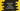# Different ways in JavaScript to check for palindrome## Introduction :

A palindrome is a sequence of characters that is the same as backward and forward. For example, rotor, level are palindrome strings.

In this post, I will show you different ways to find if a string is a palindrome or not in JavaScript. We will use different palindrome strings to check if the solutions work or not.

## Using a for loop :

This program will use one for loop to iterate through the characters of a string one by one. It will compare the start character with the end character i.e. the first character with the last character, the second character with the second last character, etc. It returns false if it will find any mismatched. Else, the loop will stop at the middle character.

``````// 1
const isPalindrome = str => {
// 2
if (str == null) {
return false;
}

// 3
const midPoint = str.length / 2;

// 4
for (let i = 0; i < midPoint && i < str.length; i++) {
if (str[i] != str[str.length - 1 - i]) {
return false;
}
}
return true;
};

const sampleStrings = [
"",
"a",
"aba",
"abba",
"ab a",
"palindrome",
"civic",
"level",
"a nut for a jar of tuna"
];

for (let item of sampleStrings) {
console.log(`\${item} : \${isPalindrome(item)}`);
}``````

### Explanation :

The commented numbers in the above program denote the step numbers below :

1. Here, isPalindrome inline function is used to check for a palindrome string. It takes one string str as its argument and returns one boolean value.
2. If str is null, return false.
3. Find the midPoint i.e. the index of the middle character. We will iterate only up to the middle character.
4. This is the for loop to iterate. We are checking if each left-side characters are equal to the right side characters or not, taking the middle index as the divider. If not, it returns false. Else, it returns true at the end.

### Output :

We are checking 10 different strings. It will print the below output :

`````` : true
a : true
aba : true
abba : true
ab a : false
palindrome : false
civic : true
level : true
a nut for a jar of tuna : false``````

This program is case sensitive and also checks for spaces. For example, the last string is a palindrome if you remove the blank spaces, but it returns false. You can remove the spaces and convert the string to lowercase if you want.

## By reversing the string :

We can simply reverse the string and compare it with the original string to find if it is a palindrome or not. Reversing is done in three steps: split the strings to create one character array, reverse the character array and join the characters to create the final reversed string.

The final program looks like as below :

``````const isPalindrome = str => {
if (str == null) {
return false;
}

return (
str ==
str
.split("")
.reverse()
.join("")
);
};

const sampleStrings = [
"",
"a",
"aba",
"abba",
"ab a",
"palindrome",
"civic",
"level",
"a nut for a jar of tuna"
];

for (let item of sampleStrings) {
console.log(`\${item} : \${isPalindrome(item)}`);
}``````

We are using the same set of strings as the first example. It will print the below output :

`````` : true
a : true
aba : true
abba : true
ab a : false
palindrome : false
civic : true
level : true
a nut for a jar of tuna : false``````

This method looks easy and concise, but note that reverse is an expensive operation and it will take more time than the first one. For production applications or for applications with large strings, the first method is more preferable.

## Recursive method :

Recursive function calls itself repeatedly until a termination point is reached. In our case, we will compare the first and the last character and then call the same palindrome check method with a substring by removing the first and the last character. This will keep running till only one or no character left or if the first and the last character are not equal.

Below is the program to find palindrome recursively :

``````const isPalindrome = str => {
if (str == null) {
return false;
}

if (str.length <= 1) return true;

if (str.charAt(0) != str.slice(-1)) return false;

return isPalindrome(str.substring(1,str.length-1));
};

const sampleStrings = [
"",
"a",
"aba",
"abba",
"ab a",
"palindrome",
"civic",
"level",
"a nut for a jar of tuna"
];

for (let item of sampleStrings) {
console.log(`\${item} : \${isPalindrome(item)}`);
}``````

Here, isPalindrome is a recursive method to check for palindrome. It returns true if the size of the string is less than or equal to 1. If the first and the last character of the string are not equal, it returns false. Else, it calls the same method recursively with a string by removing the first and the last character of the current string and returns that result.

It will print the below output :

`````` : true
a : true
aba : true
abba : true
ab a : false
palindrome : false
civic : true